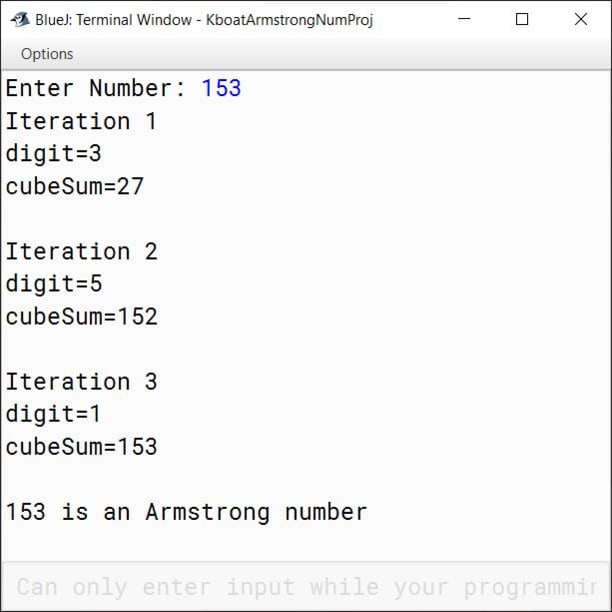PrevNextBack

# Armstrong Number Java Program

## What is an Armstrong Number?

In this lesson, we will look at Armstrong number program. A positive number is an Armstrong number if it is equal to the sum of cubes of its digits. 1, 153, 370, 371, 407 are a few examples of Armstrong number.

Taking 153 as an example:
Sum of cube of digits
= (1 x 1 x 1) + (5 x 5 x 5) + (3 x 3 x 3)
= 1 + 125 + 27
= 153

Hence, 153 is an Armstrong number as sum of cube of its digits is equal to the number itself.

## Armstrong Number Program

This is the program to check if a given number is an Armstrong number or not.

```import java.util.Scanner;

public class KboatArmstrongNum
{
public void checkArmstrong() {
Scanner in = new Scanner(System.in);
System.out.print("Enter Number: ");
int num = in.nextInt();
int orgNum = num;

int cubeSum = 0;

while (num > 0) {
int digit = num % 10;
cubeSum = cubeSum + (digit * digit * digit);
num /= 10;
}

if (cubeSum == orgNum)
System.out.println(orgNum + " is an Armstrong number");
else
System.out.println(orgNum + " is not an Armstrong number");
}
}```

Let's go over the program to understand it. We first accept the number as input from the user through Scanner class and make a copy of it.

Next, we declare an int variable `cubeSum` and initialize it to 0. We will use `cubeSum` as an accumulator to store the sum of cubes of digits.

After that we have the while loop which will run till the number is greater than 0. Inside the loop, we extract the digit, compute its cube and add it to `cubeSum`. Then we truncate the number.

After the loop, we check if sum of cubes of digits is equal to the number and print an appropriate message accordingly.

Let us add a few print statements to get some insights into what is happening in each iteration of the loop and then we will see the output and correlate it with the code.

Here is the program with the print statements added and its output:

```import java.util.Scanner;

public class KboatArmstrongNum
{
public void checkArmstrong() {
Scanner in = new Scanner(System.in);
System.out.print("Enter Number: ");
int num = in.nextInt();
int orgNum = num;

int cubeSum = 0;
int itr = 1;

while (num > 0) {
int digit = num % 10;
cubeSum = cubeSum + (digit * digit * digit);
num /= 10;
/*
* for explanation
*/
System.out.println("Iteration " + itr++);
System.out.println("digit=" + digit);
System.out.println("cubeSum=" + cubeSum);
System.out.println();
}

if (cubeSum == orgNum)
System.out.println(orgNum + " is an Armstrong number");
else
System.out.println(orgNum + " is not an Armstrong number");
}
}```

#### OutputIn the first iteration, the digit in the unit’s place which is 3 is extracted. `cubeSum` is 0, adding cube of 3 to it changes its value to 27:

cubeSum = cubeSum + (digit*digit*digit)
cubeSum = 0 + (3*3*3)    [∵ digit = 3]
cubeSum = 27

Program control goes back to the beginning of while loop and tests the condition. As number is greater than 0, 2nd iteration of while loop starts. Digit 5 is extracted from the number. Cube of 5 is 125, this gets added to `cubeSum` making it 152:

cubeSum = cubeSum + (digit*digit*digit)
cubeSum = 27 + (5*5*5)    [∵ digit = 5]
cubeSum = 27 + 125
cubeSum = 152

As number is greater than 0, 3rd iteration of while loop begins. Digit 1 is extracted. Its cube is 1 so `cubeSum` becomes 153:

cubeSum = cubeSum + (digit*digit*digit)
cubeSum = 152 + (1*1*1)    [∵ digit = 1]
cubeSum = 152 + 1
cubeSum = 153

Again, program control goes back to the beginning of while loop. But now the condition becomes false as number is 0. Program control comes to the if check after the while loop. It compares the number with the sum of cubes of its digits. For 153, both are equal so 153 is an Armstrong number gets printed to the console.